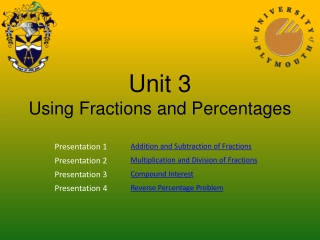DownloadDownload PresentationUnit 3 Using Fractions and Percentages

# Unit 3 Using Fractions and Percentages

Download Presentation## Unit 3 Using Fractions and Percentages

- - - - - - - - - - - - - - - - - - - - - - - - - - - E N D - - - - - - - - - - - - - - - - - - - - - - - - - - -
##### Presentation Transcript

1. Unit 3Using Fractions and Percentages

2. Unit 3Using Fractions and Percentages Addition and Subtraction of Fractions

3. When adding or subtracting fractions, you must have the same denominator. Example: Lowest common multiple of 4 and 5 is 20 (or )

4. When adding or subtracting fractions, you must have the same denominator. Example:

5. You have finished viewing the presentationAddition and Subtraction of FractionsPlease choose an option

6. Unit 3Using Fractions and Percentages Multiplication and Division of Fractions

7. The rule is: Example: 3 is a common factor in both the numerator and denominator

8. The rule is: Example:

9. Example: First Calculate Thus

10. You have finished viewing the presentationMultiplication and Division of FractionsPlease choose an option

11. Unit 3Using Fractions and Percentages Compound Interest

12. How much is \$140 worth after 10 years in an account that pays 5% interest per year When An is the total amount after investing A0 for n years at r%

13. You have finished viewing the presentationCompound InterestPlease choose an option

14. Unit 3Using Fractions and Percentages Reverse Percentage Problem

15. The price of a computer is \$1398, including GCT at What was the price of the computer without GCT? To add 16.5% GCT, you multiply by 1.165 To remove GCT, you divide by 1.165 Computer price

16. You have finished viewing the presentationReverse Percentage ProblemPlease choose an option